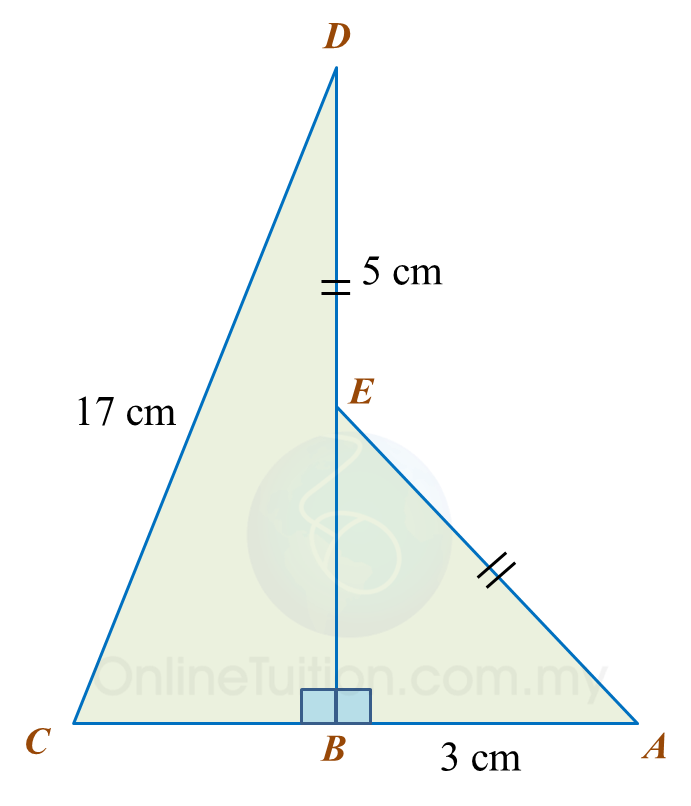# 13.2.4 The Pythagoras’ Theorem, PT3 Focus Practice

  
      
      
Question 8:
      
In diagram below ABDE is a square and EDC is a straight line.The area of the square ABDE is 144 cm2.
      
Calculate the length, in cm, of BC.
      
      

    Solution:
      
BD = √144
      
= 12 cm
      
BC= 122 + 92
      
 = 144 + 81
      
 = 225
      
BC = √225
      
= 15 cm
  
  
 
 
Question 9:
Diagram below shows two right-angled triangles, ABE and CBD. BED is a straight line.Find the length, in cm, of BC. Round off the answer to two decimal places.

Solution:

Question 10:
Diagram below shows two right-angled triangles, ABC and ADE. ACD is a straight line.Find the length, in cm, of AE. Round off the answer to one decimal places.

Solution: